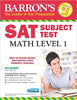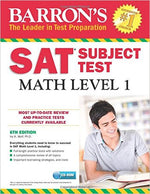SAT SUBJECT MATH LEVEL 1
Review: 5 - "A masterpiece of literature" by , written on May 4, 2006
I really enjoyed this book. It captures the essential challenge people face as they try make sense of their lives and grow to adulthood.# SAT SUBJECT MATH LEVEL 1

Available: Out of Stock
12.000 BHD
Successful pre-order.Thanks for contacting us!
• ##### SAT SUBJECT MATH LEVEL 112.000 BHD
This product: SAT SUBJECT MATH LEVEL 1
12.000 BHD 1200
12.000 BHD

This new edition of Barron's SAT Subject Test: Math Level 1 offers students intensive test preparation with:

A review of the most important test-taking strategies students need to know to succeed on this exam

Topic review chapters covering all the math students need to know for this test, including: arithmetic, algebra, plane geometry, solid and coordinate geometry, trigonometry, functions and their graphs, probability and statistics, real and imaginary numbers, and logic

Three full-length model tests with complete solutions for every problem

The manual can be purchased alone or with an optional CD-ROM that presents two additional full-length practice tests with answers, explanations, and automatic scoring.

From the Inside Flap

What You Need to Know About SAT Subject Tests

What You Need to Know About the SAT Subject Test in Math Level 1

TEST-TAKING STRATEGIES

Important Tactics

MATH REVIEW

Basic Arithmetic

Fractions, Decimals, and Percents

Ratios and Proportions

ALGEBRA

Polynomials

Equations and Inequalities

Word Problems

PLANE GEOMETRY

Lines and Angles

Triangles

Polygons

Circles

SOLID AND COORDINATE GEOMETRY

Solid Geometry

Coordinate Geometry

TRIGONOMETRY

Basic Trigonometry

FUNCTIONS

Functions and Their Graphs

STATISTICS, COUNTING, AND PROBABILITY

Basic Concepts of Statistics, Counting, and Probability

MISCELLANEOUS TOPICS

Imaginary and Complex Numbers

Sequences

Logic

MODEL TESTS

Guidelines and Scoring

Model Test 1

Model Test 2

Model Test 3

Index

Product Details

Paperback: 408 pages

Publisher: Barron's Educational Series; 6 edition (September 1, 2016)

Language: English

ISBN-10: 1438076312

ISBN-13: 978-1438076317

Product Dimensions: 8.3 x 0.7 x 10.8 inches

This new edition of Barron's SAT Subject Test: Math Level 1 offers students intensive test preparation with:

A review of the most important test-taking strategies students need to know to succeed on this exam

Topic review chapters covering all the math students need to know for this test, including: arithmetic, algebra, plane geometry, solid and coordinate geometry, trigonometry, functions and their graphs, probability and statistics, real and imaginary numbers, and logic

Three full-length model tests with complete solutions for every problem

The manual can be purchased alone or with an optional CD-ROM that presents two additional full-length practice tests with answers, explanations, and automatic scoring.

From the Inside Flap

What You Need to Know About SAT Subject Tests

What You Need to Know About the SAT Subject Test in Math Level 1

TEST-TAKING STRATEGIES

Important Tactics

MATH REVIEW

Basic Arithmetic

Fractions, Decimals, and Percents

Ratios and Proportions

ALGEBRA

Polynomials

Equations and Inequalities

Word Problems

PLANE GEOMETRY

Lines and Angles

Triangles

Polygons

Circles

SOLID AND COORDINATE GEOMETRY

Solid Geometry

Coordinate Geometry

TRIGONOMETRY

Basic Trigonometry

FUNCTIONS

Functions and Their Graphs

STATISTICS, COUNTING, AND PROBABILITY

Basic Concepts of Statistics, Counting, and Probability

MISCELLANEOUS TOPICS

Imaginary and Complex Numbers

Sequences

Logic

MODEL TESTS

Guidelines and Scoring

Model Test 1

Model Test 2

Model Test 3

Index

Product Details

Paperback: 408 pages

Publisher: Barron's Educational Series; 6 edition (September 1, 2016)

Language: English

ISBN-10: 1438076312

ISBN-13: 978-1438076317

Product Dimensions: 8.3 x 0.7 x 10.8 inches